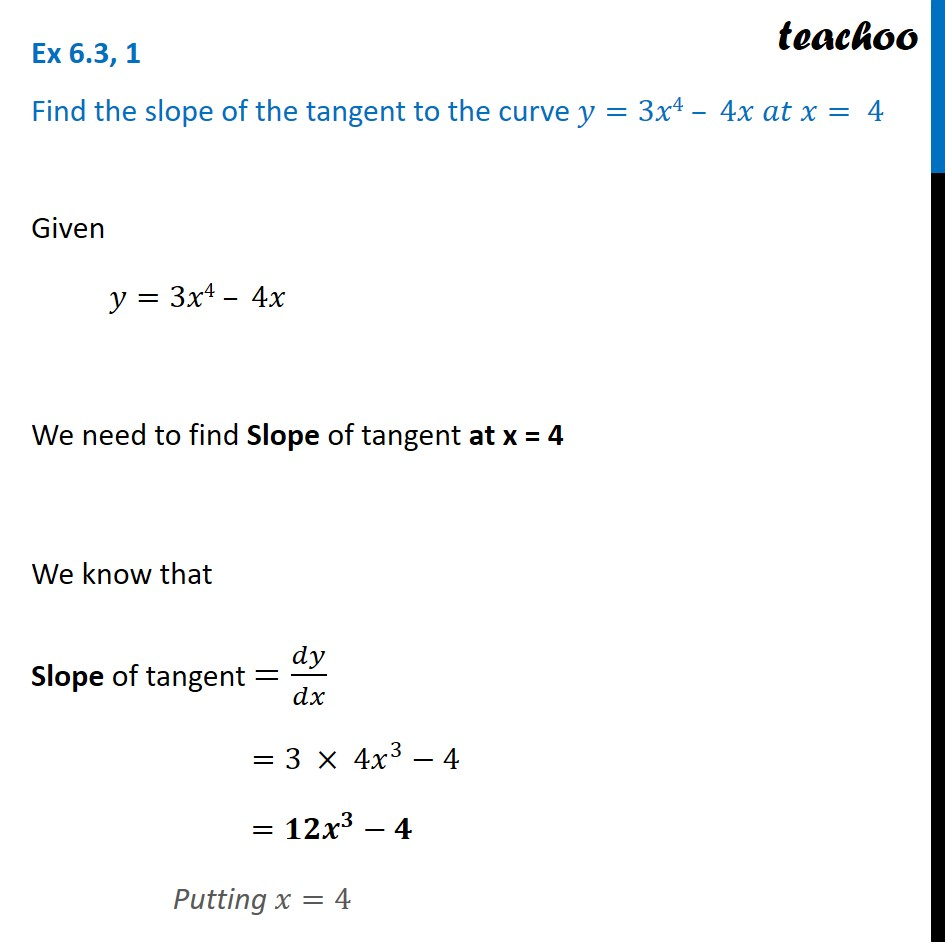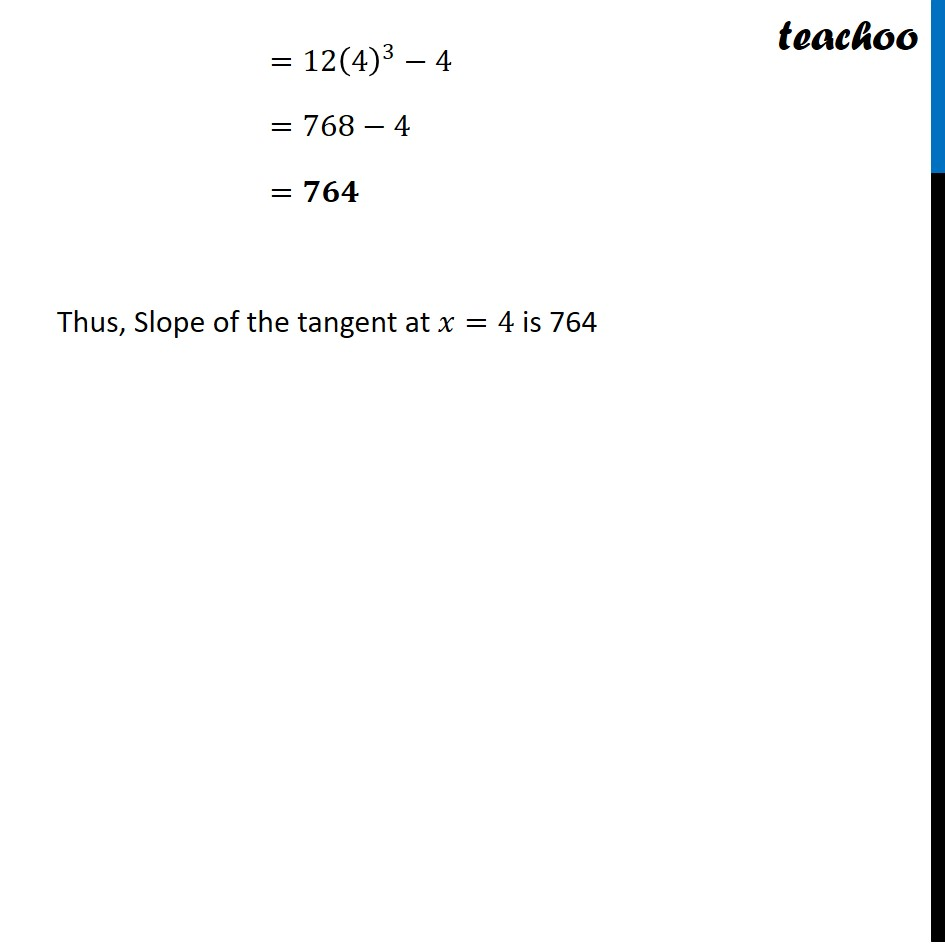Tangents and Normals (using Differentiation)

Chapter 6 Class 12 Application of Derivatives
Serial order wiseLearn in your speed, with individual attention - Teachoo Maths 1-on-1 Class

### Transcript

Question 1 Find the slope of the tangent to the curve 𝑦=3𝑥4 – 4𝑥 𝑎𝑡 𝑥= 4Given 𝑦=3𝑥4 – 4𝑥 We need to find Slope of tangent at x = 4 We know that Slope of tangent =𝑑𝑦/𝑑𝑥 =3 × 4𝑥^3−4 =𝟏𝟐𝒙^𝟑−𝟒 Putting 𝑥=4 =12(4)^3−4 =768−4 =𝟕𝟔𝟒 Thus, Slope of the tangent at 𝑥=4 is 764